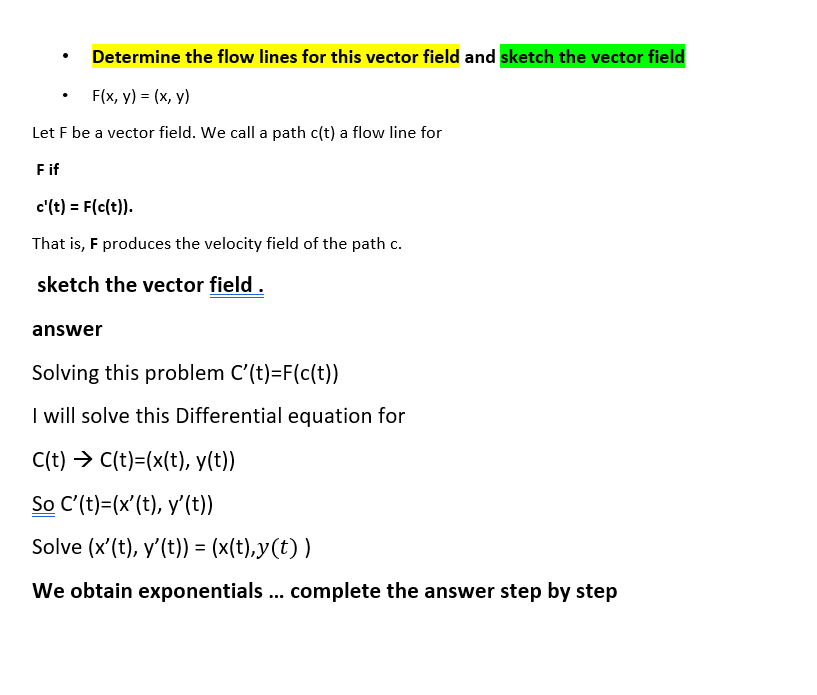### Create an Account

Already have account?

### Forgot Your Password ?

Home / Questions / Determine the flow lines for this vector field and sketch the vector field F(x, y) = (x, y...

# Determine the flow lines for this vector field and sketch the vector field F(x, y) = (x, y) Let F be a vector field. We call a path c(t) a flow line for Fif c'(t) = F(c(t)). That is, F produces the ve

Determine the flow lines for this vector field and sketch the vector field F(x, y) = (x, y) Let F be a vector field. We call a path c(t) a flow line for Fif c'(t) = F(c(t)). That is, F produces the velocity field of the path c. sketch the vector field. answer Solving this problem C'(t)=F(c(t)) I will solve this Differential equation for C(t) = C(t)=(x(t), y(t)) So C'(t)=(x'(t), y'(t)) Solve (x"(t), y'(t)) = (x(t),y(t)) We obtain exponentials ... complete the answer step by stepApr 14 2021 View more View Less

#### Answer (Solved)Subscribe To Get Solution